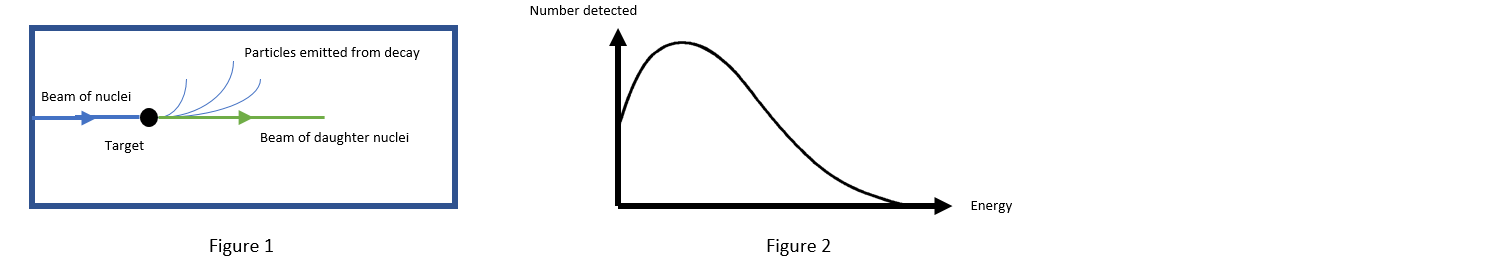Problem: As shown in figure 1, a beam of particles is fired at a stationary target. The resulting nuclei from this collision are highly unstable, and decay almost immediatebly into more stable daughter nuclei. During this decay, charged particles are emitted, which curve in the magnetic field within the detector (in this case, the field is pointing out of the page). Each of these decay particles are collected by the detector and their energies are measured, producing the graph shown in figure 2. What type of decay are the unstable nuclei undergoing? A) α decay B) β- decay C) β+ decay D) γ decay

87% (80 ratings)
Problem Details

As shown in figure 1, a beam of particles is fired at a stationary target. The resulting nuclei from this collision are highly unstable, and decay almost immediatebly into more stable daughter nuclei. During this decay, charged particles are emitted, which curve in the magnetic field within the detector (in this case, the field is pointing out of the page). Each of these decay particles are collected by the detector and their energies are measured, producing the graph shown in figure 2. What type of decay are the unstable nuclei undergoing?

A) α decay

B) β- decay

C) β+ decay

D) γ decay